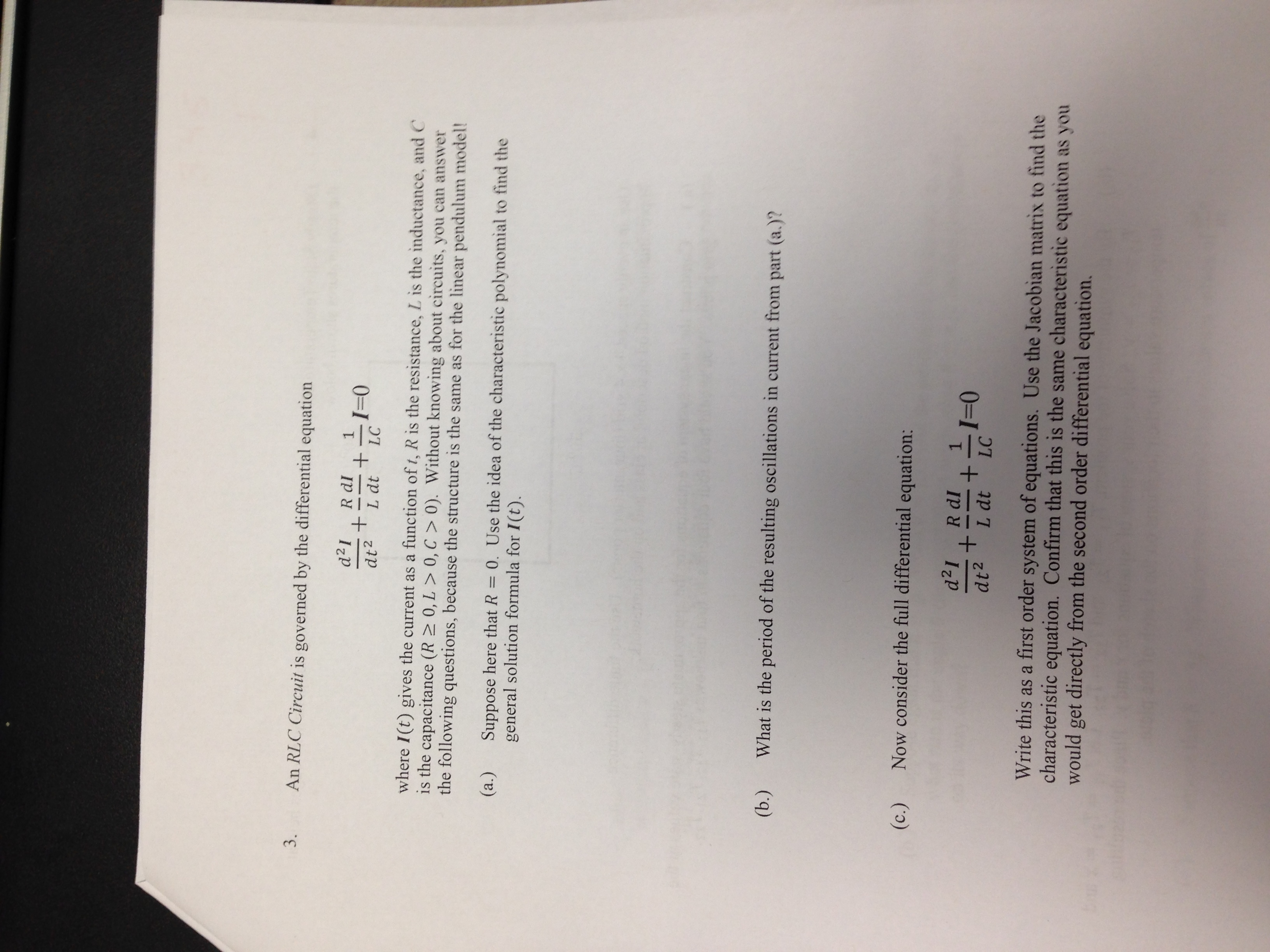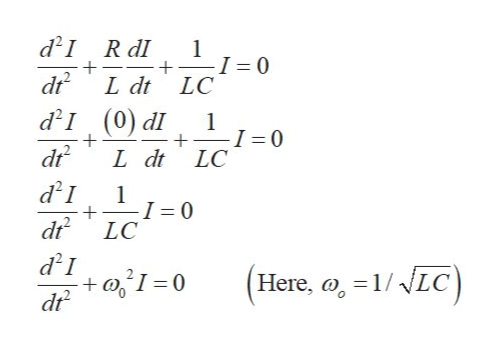# 3.An RLC Circuit is governed by the differential equationd21R dl+I-0dt2L dtLCwhere I(t) gives the current as a function of t, R is the resistance, L is the inductance, and Cis the capacitance (R2 0, L > 0, C> 0). Without knowing about circuits, you can answerthe following questions, because the structure is the same as for the linear pendulum model!(a.)Suppose here that R 0. Use the idea of the characteristic polynomial to find thegeneral solution formula for I(t).What is the period of the resulting oscillations in current from part (a.)?(b.)Now consider the full differential equation:(c.)d21R dl+dt2+I-0L dtLCWrite this as a first order system of equations. Use the Jacobian matrix to find thecharacteristic equation. Confirm that this is the same characteristic equation as youwould get directly from the second order differential equation.

Question
15 viewshelp_outlineImage Transcriptionclose3. An RLC Circuit is governed by the differential equation d21 R dl +I-0 dt2 L dt LC where I(t) gives the current as a function of t, R is the resistance, L is the inductance, and C is the capacitance (R2 0, L > 0, C> 0). Without knowing about circuits, you can answer the following questions, because the structure is the same as for the linear pendulum model! (a.) Suppose here that R 0. Use the idea of the characteristic polynomial to find the general solution formula for I(t). What is the period of the resulting oscillations in current from part (a.)? (b.) Now consider the full differential equation: (c.) d21 R dl + dt2 +I-0 L dt LC Write this as a first order system of equations. Use the Jacobian matrix to find the characteristic equation. Confirm that this is the same characteristic equation as you would get directly from the second order differential equation. fullscreen
check_circle

Step 1

(a)Write the given differential equation and plug 0 for R, we gethelp_outlineImage Transcriptionclosed21 R dI 1 I 0 LC dt L dt d2I (0) d dr I 1 -I = 0 + L dt LC d21 1 I 0 LC d21 +I0 dr (Here, o, 1/NLC fullscreen
Step 2

The characteristic equation can be given as,

Step 3

Hence, the general solution...

### Want to see the full answer?

See Solution

#### Want to see this answer and more?

Solutions are written by subject experts who are available 24/7. Questions are typically answered within 1 hour.*

See Solution
*Response times may vary by subject and question.
Tagged in

### Science